###### byAleksey Tatsitov

Choosing of assets for trading can take a long time, especially when it comes to options trading. Keeping this in mind, we’ve created the options screener that saves your time and helps find new trading ideas based on a simple principle – buying cheap options or selling expensive ones.

In this article we’ll talk about time decay role in the profitability of the vertical spread strategies. We’ll discuss why a directional movement is often not enough to generate significant profits when trading vertical spreads. Additionally, we’ll consider how extrinsic value influences the prices of vertical spreads, and how the decrease in extrinsic value is a key component to profiting when trading vertical spreads.

## The Nature of Intrinsic and Extrinsic Value of Vertical Spreads

How does time decay or the decrease in extrinsic value as time passes play a role in the performance of the vertical spread strategies?

The extrinsic value related to vertical spreads means that for a vertical spread to reach the maximum profit potential or for the spread to reach the price that will realize the maximum profit potential the extrinsic value of the options in that spread must reach zero dollars.

Let’s go ahead and start with a hypothetical example. Let’s say the stock price is currently at \$163.91 and we go ahead and look at the Long 160 / 162.5 call spread. The spread price is \$1.68. But we know that the spreads value could rise to \$2.50 if it is fully in-the-money at the time of expiration because a spread can only be worth as much as the width of the strikes. In this case, the strikes are \$2.50 apart and therefore the maximum value that this spread can reach is \$2.50

Since this long vertical call spread is fully in-the-money and we know it can be worth \$2.50 at a maximum why isn’t the spread currently trading for \$2.50 if it’s fully in-the-money? The answer is that both options still have extrinsic value remaining.

Suppose the 160 call is worth \$5.88 with \$3.91 of an intrinsic value and a \$1.97 of extrinsic value, and the 162.5 call is worth \$4.20 with \$1.41 of an intrinsic value and a \$2.79 of extrinsic value. As we can see both of the options still have plenty of extrinsic value remaining. When we calculate the price of the long call spread with intrinsic value only we can see that the spreads value is \$2.50 which is the width of these strikes.

Since the 160 call has \$3.91 of intrinsic value and the 162.5 short call, in this case, has a \$1.41 of intrinsic value if you take \$3.91 and subtract \$1.41 you’ll get \$2.50. So just including intrinsic value, our spread is worth 2.50 of intrinsic value.

As we’ve seen before the call spread is currently trading for a \$1.68 and as we’ve discovered both of these options have lots of extrinsic value remaining.

What is going to happen between now and expiration if the stock price stays right at \$163.91? The answer is that these options are going to decrease in value and at expiration they will only reflect the intrinsic value of the options, so if the stock price stays right at the same mark the only thing that’s going to happen between now and expiration is the decrease in extrinsic value which we call time decay.

This is important because when trading vertical spreads the passage of time is a critical component to reaching high-profit levels as option prices trade with less and less extrinsic value as they approach expiration.

This is important to understand because when you trade vertical spreads, you’ll typically start by focusing on the directional aspect of that spread at least initially. For example, if you buy a call spread you want the stock price to increase and ideally, you want the stock price to blow through your call strikes because you know that’s going to generate profits for your position.

However you also need to consider the extrinsic value or the amount of time remaining until that spread expires because if you get that direction of movement that you’re willing you might not have the profits that you thought you were going to have, because those options are still going to have a lot of extrinsic value if they expire in a couple weeks or a couple months. Therefore, it’s necessary to discuss how to choose an expiration cycle.

## Extrinsic Value and Vertical Spread Profit/Loss Estimation in Real Options

Let’s take some examples to demonstrate profit/loss estimations based on the passage of time and the movement in the stock price as they relate to the vertical spread strategies.

Let’s look at Apple options in October of 2017. The options have 64 days to expiration and Apple shares are trading around \$160. Let’s start by looking at the 155/170 bull call spread. As you know the bull call spread means you’re buying a call option and then selling another call at a higher strike price in the same expiration cycle.

For this example, I’m going to look at buying the 155 call and then also selling the 170 call against it and that is going to create our call vertical spread using the 155 and 170 call options (Figure 1).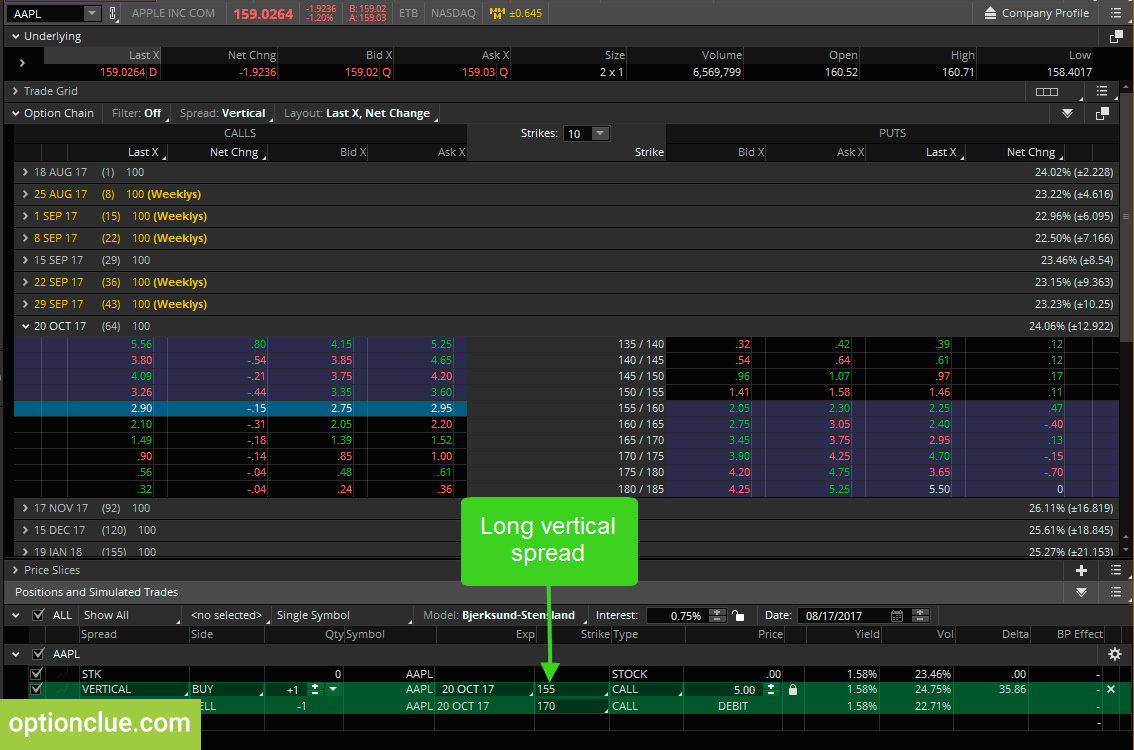This spread is trading for \$5.50. In the risk profile, we can see two different lines (Figure 2). This blue line is the expiration pay off line; this is what is the profit/loss of the spread going to be based on Apple’s price at expiration.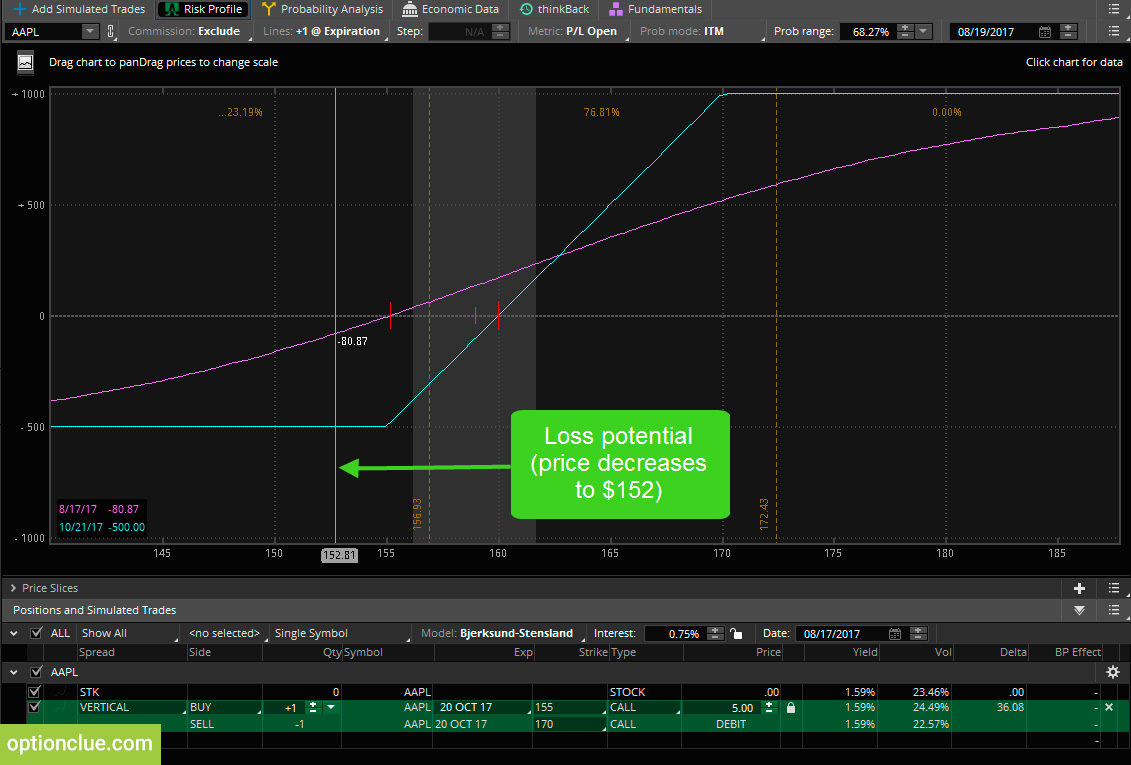Since this is a bull call spread, if the stock price is at or below the long call strike price at expiration we will lose the maximum amount, which is the exact amount that we’re paying for the spread. Since we paid \$5 for this spread if Apple is at or below \$155 at expiration, we will lose \$500 per spread (the lower left-hand corner of figure 2).

The pink line is the profit/loss estimation of this spread based on Apple’s share price today. Let’s say Apple fell to \$152 per share today. Based on that move this profit/loss estimation is showing that we would lose \$80 per call spread. That’s good news because that means if you buy a call spread and the stock price moves against you quickly you’re not going to have the maximum loss potential of that spread.

Based on the graph this is saying that if the stock price goes to 155 we expect to lose about 80 dollars on this spread but if Apple is at 155 or below at expiration we know we will lose \$500 so the loss is minor if the stock price moves against you initially.

The bad news is that since we paid \$5 for this \$15 widespread the maximum profit potential at expiration is \$1000 (Figure 3).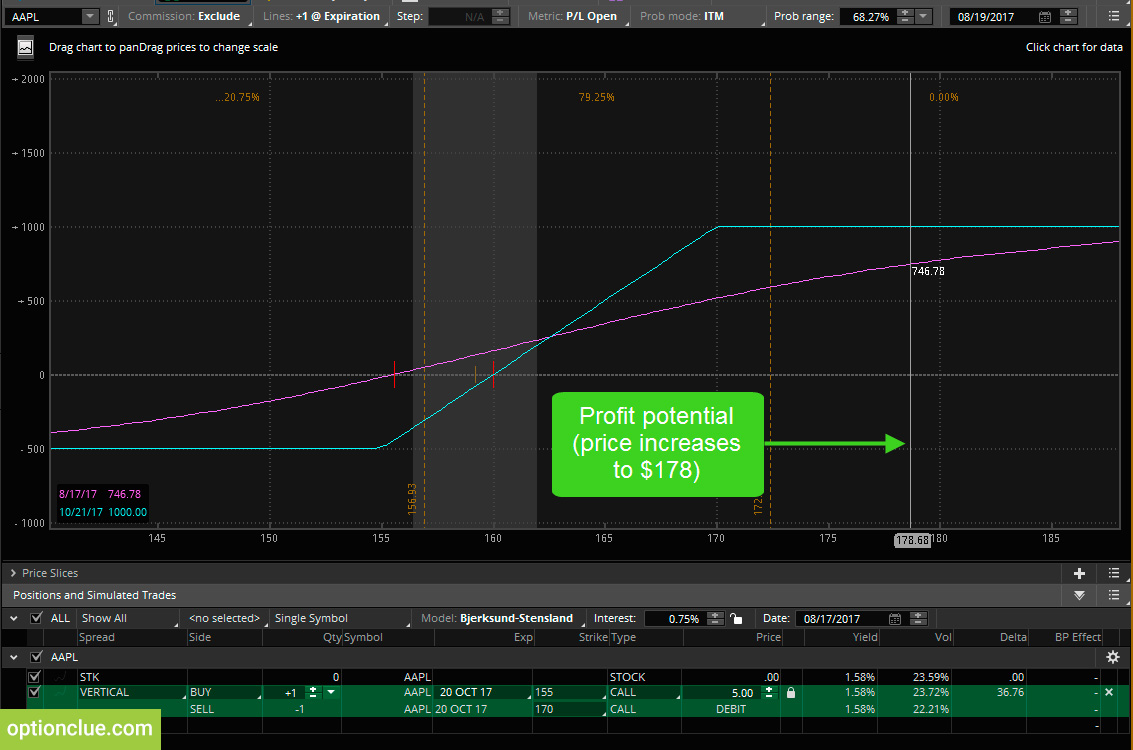That occurs if Apple is at or above \$170 at expiration. As we can see the lower left-hand corner says that at any price above 170 the profit is going to be \$1000. However let’s say you bought this call spread today and Apple’s at \$160 and it shoots all the way up to \$172 today, that pink line says that we only expect to profit about \$746.

That means if the stock price moves above the short call strike price we’re going to be up about 3/4 of the profit potential that this position has. The reason for that is because we know that we need this spread to lose its extrinsic value. To reach that 1000 profit target we need Apple to move up to 170 or higher but we also need time to pass.

### Time Decay and Vertical Spread Profit/Loss Estimation

Suppose we were one week before expiration (Figure 4). As we can see, this pink line is now much closer to the expiration pay off line and that’s because one week before expiration these options are going to have much less extrinsic value.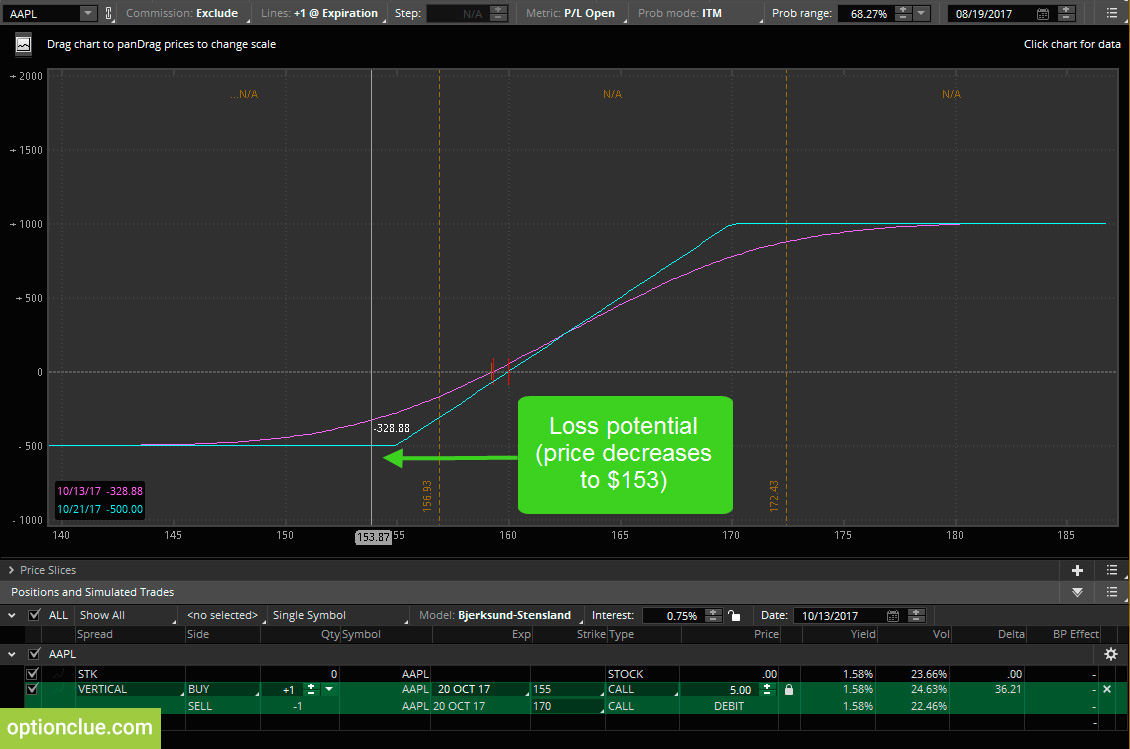Figure 4. Call vertical spread. Loss. One week before expiration (thinkorswim trading platform)

if the stock price moved to \$152 it was estimated that we would be down an \$80 today but if Apple is at a \$153 with about a week to go into expiration, this pink line is saying that we’d be down about \$328. Closer to expiration at the same price we’re down more money because there’s less extrinsic value in these options.

On the other side if Apple is at 170 with a week to go before expiration (Figure 5) this estimator is saying that will be up around \$866, less than in the previous example.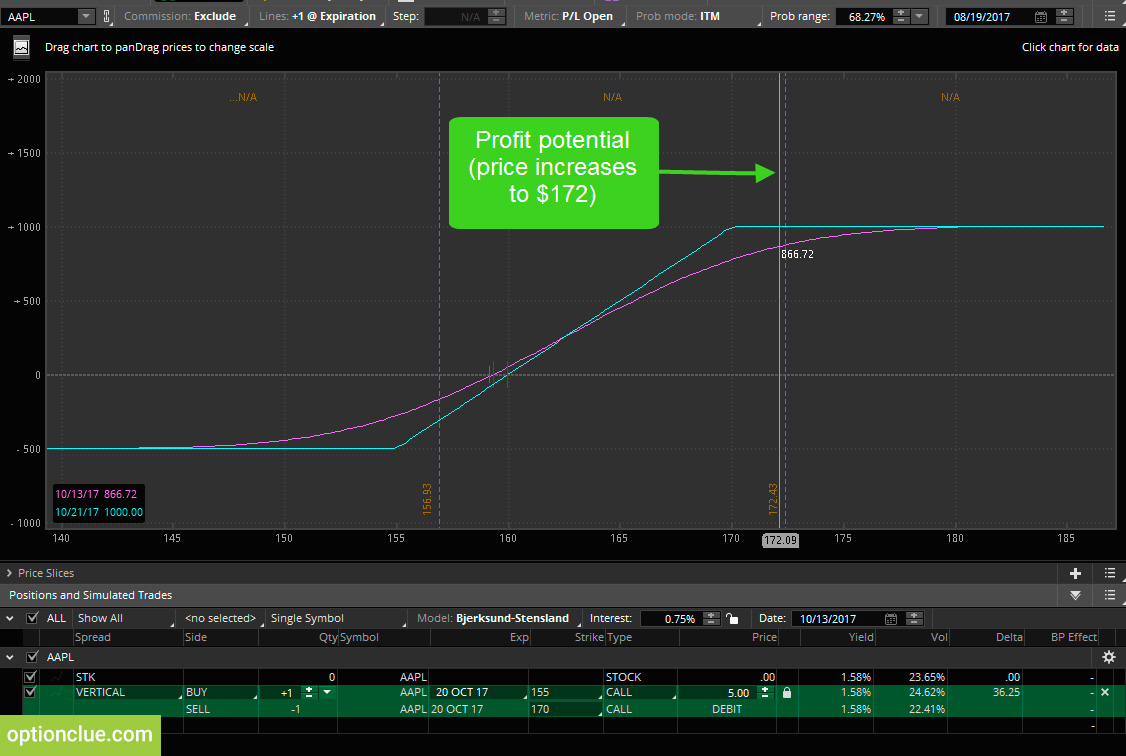Figure 5. Call vertical spread. Profit. One week before expiration. (thinkorswim trading platform)

This just shows that when you trade vertical spreads you want the stock price to move in favor of your spread, but you also need time to pass because as time passes extrinsic value is traded out of the options and that means you will have a higher probability of reaching the higher profit targets for your spread.